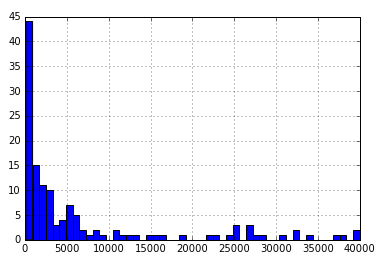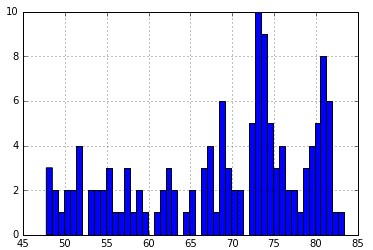# Making Data Management Decisions

2 minutes read

As part of the Data Management and Visualisation course on Coursera, I am investigating the following hypothesis from the Gapminder dataset

What effect does the “level of democracy” in a country have on the “quality of life” of the country’s citizens?

In the previous extrapolatory analysis completed last week, I decided that I would be measuring the correlation between the “polityscore” (a numeric value from -10 to 10 that measures how democratic a country is)variable and the following variables in the dataset

• incomeperperson(2010 GDP per capita in constant US\$ 2000)

• alconconsumption(2008 alcohol consumption per adult)

• breastcancerper100TH(breast cancer new cases per 10,000)

• employrate(2007 total emloyees over 15+)

• HIVrate(2009 estimated HIV prevalence)

• lifeexpectancy(2011 life expectancy at birth)

• urbanrate(Urban population)

However, I want to ensure that I am only reading data for countries from the dataset that do not have any missing values. For this, I can the following code snippet, which only subsetted the values from the dataset that were complete for all these variables.

This code also converted the variables to numeric values, since they would be required for calculating the correlation between the variables

``````import pandas as pd
import numpy as np
data = pd.read_csv(‘gapminder.csv’, low_memory=False)
#setting variables you will be working with to numeric
data[‘incomeperperson’] = data[‘incomeperperson’].convert_objects(convert_numeric=True)
data[‘alcconsumption’] = data[‘alcconsumption’].convert_objects(convert_numeric=True)
data[‘breastcancerper100th’] = data[‘breastcancerper100th’].convert_objects(convert_numeric=True)
data[‘employrate’] = data[‘employrate’].convert_objects(convert_numeric=True)
data[‘hivrate’] = data[‘hivrate’].convert_objects(convert_numeric=True)
data[‘lifeexpectancy’] = data[‘lifeexpectancy’].convert_objects(convert_numeric=True)
data[‘urbanrate’] = data[‘urbanrate’].convert_objects(convert_numeric=True)
data[‘polityscore’] = data[‘polityscore’].convert_objects(convert_numeric=True)
#select data subset that does not have any missing polity scores
subset = data[np.isfinite(data[‘polityscore’])]
subset = subset[np.isfinite(subset[‘incomeperperson’])]
subset = subset[np.isfinite(subset[‘alcconsumption’])]
subset = subset[np.isfinite(subset[‘breastcancerper100th’])]
subset = subset[np.isfinite(subset[‘employrate’])]
subset = subset[np.isfinite(subset[‘hivrate’])]
subset = subset[np.isfinite(subset[‘lifeexpectancy’])]
subset = subset[np.isfinite(subset[‘urbanrate’])]
``````

I shall not be grouping any of the variables, since at this time in my analysis, I do not see the need of doing it.

I generated a frequency table for the polityscores, using the following code snippet.

``````group = subset.groupby(‘polityscore’).size()
print group
``````

The display was as follows

However, since the other variables are all continuous variables, creating frequency tables for them does not make sense. Rather, their distributions and frequencies can be showcased via histograms.

As an example, the following code snippet displays the histogram for the distribution of income distributions across the dataset.

``````subset[‘incomeperperson’].hist(bins=50)
``````

The following is the histogram for incomeperperson displayedHistogram for Income per person across the dataset

The following code was used to display a histogram for life expectancy rates

``````subset[‘lifeexpectancy’].hist(bins=50)
``````

A frequency table for the polityscore variable was achieved with the following

``````group = subset.groupby(‘polityscore’).size()
print group
``````

The display was as followsHistogram for Distribution of Life Expectancy

As can be seen from the above histogram, the life expectancy varies more across the dataset, which hints at the fact that there are more factors that determine the life expectancy of a country rather than just how “democratic” it is.

This post was originally published on Medium